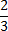Study Guide

# Ratios & Percentages - Converting Fractions to Percents

## Converting Fractions to Percents

Next up on this crazy conversion train: transforming fractions into percents. If a fraction can be written with a denominator of 100, converting it to a percent is easy peasy.

Take a gander at the image below.Two fifths can be converted to forty hundredths by multiplying the top and bottom by 20.

2 × 20 = 40
5 × 20 = 100

Because all percents are out of 100, this can be written as 40%.

Just like with converting fractions to decimals, this technique doesn't always work. The good news is that there are no new steps to learn. Convert the fraction to a decimal, then the decimal to a percent.

For example, a fraction likecan't be converted into a fraction with 100 in the denominator, because 3 doesn't go into 100 evenly. Here's what we'll do instead.

Step 1: turn the fraction into a decimal. Using our trick for turning fractions into decimals, we divide the numerator by the denominator.

2 ÷ 3 = 0.66666...

Step 2: turn the decimal into a percent. Using our trick for turning decimals into percents, we multiply the decimal by 100. In other words, move the decimal point to the right by two places.

0.66666... × 100 = 66.66666...

We'd rather not write out an infinite number of 6s, so we'll round that to 66.67%. That percentage is the same thing as the fraction.